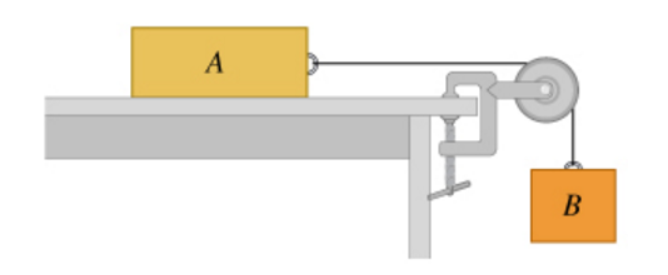# Problem: Consider the system shown in the figure (Figure 1). Block A weighs 46.9 N and block B weighs 30.1 N.Once block B is set into downward motion, it descends at a constant speed.a.) Calculate the coefficient of kinetic friction between block A and the tabletop.b.) A cat, also of weight 46.9 N, falls asleep on top of block A. If block B is now set into downward motion, what is its acceleration magnitude?

###### FREE Expert Solution

The kinetic frictional force:

$\overline{){{\mathbf{F}}}_{{\mathbf{f}}}{\mathbf{=}}{{\mathbf{\mu }}}_{{\mathbf{k}}}{\mathbf{N}}}$

The normal force:

$\overline{){\mathbf{N}}{\mathbf{=}}{\mathbf{m}}{\mathbf{g}}}$

a)

The sum of horizontal forces on A is:

ΣFA = T - fA

0 = T - μkmAg

The sum of horizontal forces acting on B:

ΣFB = WB - T

0 = WB - T = mBg - T

84% (288 ratings)###### Problem Details

Consider the system shown in the figure (Figure 1). Block A weighs 46.9 N and block B weighs 30.1 N.Once block B is set into downward motion, it descends at a constant speed.a.) Calculate the coefficient of kinetic friction between block A and the tabletop.

b.) A cat, also of weight 46.9 N, falls asleep on top of block A. If block B is now set into downward motion, what is its acceleration magnitude?